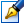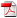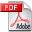##The generalized complex of multi-ary relations and its applicative aspects

 Author: Cataranciuc Sergiu Degree: doctor habilitat of physics and mathematics Speciality: 01.01.09 - Mathematical cybernetics and operation research Year: 2015 Scientific consultant: Petru Soltandoctor habilitat, professor, Moldova State University Institution: Moldova State University

### Status

The thesis was presented on the 2 July, 2015
Approved by NCAA on the 7 October, 2015

### Abstract1.03 Mb / in romanian

### Thesis

CZU 519.1+515.142.215.59 Mb / in romanian
289 pages

### Keywords

Complex of multi-ary relations, discrete optimization, homology groups, abstract cube, quasi simplex, Euler-Poincare characteristic, metric space, convexity, abstract manifolds, a median, Grundy function

### Summary

ANNOTATION of the thesis “The generalized complex of multi-ary relations and its applicative aspects”, prezented by Cataranciuc Sergiu for obtaining the Doctor Habilitat degree in Mathematics, specialty 112.03 – Mathematical Cybernetics and Operations Research The thesis has been elaborated in Chişinău, Moldova State University, in 2015. Thesis structure: The thesis is written in Romanian and contains an introduction, five chapters, general conclusions and recommendations, a bibliography of 309 titles and 12 figures. The results are published in 58 scientific papers. Keywords: Complex of multi-ary relations, discrete optimization, homology groups, abstract cube, quasi simplex, Euler-Poincare characteristic, metric space, convexity, abstract manifolds, a median, Grundy function. Field of study of the thesis: Discrete optimization. The aim of research: Development of the discrete mathematical structures and effective methods for modeling and solving the problems of placing the median, the center, as well as their different variations; elaboration and development of the new mathematical model based on multi-ary relations defined on the Cartesian product of a set of elements; the study of the topology of multi-ary relations with the help of discrete structures named a complex of multi-ary relations; the studying the complex of abstract cubes, as a particular case of the complex of multi-ary relations and the corresponding manifold; the development of an efficient algorithm for solving the median problem on a complex of abstract cubes; the generalization of Grundy function followed by its application to combinatorial games on a complex of multi-ary relations; The scientific novelty and originality is reflected in the following: it was proposed a new direction for research conditioned by the necessity of study the properties of complex of multi-ary relations and its application for solving discrete optimization problems; it was derived recursively formula for calculating cyclomatic number for the complex of multi-ary relations using the ranks of the homology groups; it was generalized the concept of d-convexity for metric spaces defined by k-ary relations, ≤k≤n, , of the complex Rn+1 =( R¹, R²,..., Rn+1), it was developed an algorithm for calculating the median of the complex of abstract cubes. Fundamentally new results for science and practice: It was identified and examined a new mathematical structure - the complex of multi-ary relations. The methods and structures of algebraic topology involving the homology groups have been used. The method for determining the median without the use of the space metrics has been elaborated. New direction proposed for research: The development of the theory of complex of multi-ary relations, that generalizes a number of classical discrete structures, based on which are developed new models and methods for their continued use to solve the discrete optimization problems. The theoretical significance of the work is determined by the foundation of a new direction for research, generated by the study of algebraic topology of multi-ary relations. To solve discrete optimization problems, the new mathematical structure that generalizes the classical known discrete structures have been proposed. The applicative value of the paper: The method of calculating the median of the complex of abstract cubes without the use of the space metrics has been elaborated. Using the generalized Grandy function, the method for solving combinatorial games has been proposed. The implementation of scientific results: The results might provide a basis for selecting the themes for doctoral studies. Also they may be employed in the educational process at the faculty of Mathematics and Computer Science as well as for solving some real economic problems.